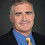## Thursday, January 09, 2014

### Is Traveling Salesman NP-Complete?

[Nina Balcan asked me to mention that the COLT submission deadline is February 7]

Jean Francois Puget writes a controversial post No, The TSP Isn't NP Complete which I discovered during a lengthy twitter discussion with Puget and Peter Cacioppi.

There is a well-known technicality for the Euclidean Traveling Salesman problem but let's focus instead where we are given a complete graph weighted with positive integers. One version of TSP is truly NP-complete
TSP Decision: Given an integer B, is there a cycle through all the vertices such that the total weight of the edges used is at most B?
TSP Decision is in NP by guessing the cycle and hardness by a simple reduction from Hamiltonian Cycle.

Puget's makes the point that we normally think of the TSP problem as an optimization question
TSP Minimization: Find the cycle through all the vertices that minimizes the total weight used.
TSP Minimization is not even a decision problem. In the 80's, Mark Krentel created a complexity class OptP to capture optimization problems and showed that TSP Minimization is Opt-P-complete.

One can use TSP Decision to solve TSP minimization by doing binary search, so they have effectively have the same complexity.

Puget points out that even if we are given a tour, checking that it is the shortest tour is not believed to be in NP. That problem is in co-NP and I'm guessing co-NP-complete. [Update 3/20: I was right]

Puget doesn't like when people claim TSP is NP-complete when they are talking about the optimization problem. For example from my P v NP survey
The NP-complete traveling salesperson problem asks for the smallest distance tour through a set of specified cities.
I'm far less bothered than Puget. Those who understand the technicalities of NP-completeness know that one has to convert the optimization problem to an appropriate decision problem to formally get an NP-complete set. Others aren't led too far astray, for we do have an equivalence that P = NP if and only if there is an efficient (polynomial-time) algorithm for TSP Minimization.

1."Puget points out that even if we are given a tour, checking that it is the shortest tour is not believed to be in NP. That problem is in co-NP"

Can you prove that it is in co-NP? Wouldn't that require deciding "Is this path shorter than that path?" in polynomial time? Isn't that just just SQRT-SUM in disguise, and therefore not known to be in P?

2.The co-NP certificate is just a path of shorter length. All you have to do is verify it's a path and add up its weights. Certainly polynomial time.

3.This comment has been removed by the author.

4."That problem is in co-NP..."

That statement wasn't about the Euclidean TSP, was it? :)

5.Ah, I see. The co-NP comment was about the "explicit edge weights" case, even though it is refering to a statement by Puget, who only talks about the Euclidean case. My mistake.

1.I did not talk about the Euclidian case, for the record.

6.The biggest problem might be that some PROFESSORS don't understand how to word TSP as an NP-complete problem. I'm not talking about the level of professors reading and writing here for the most part, but rather than at many of the thousands of colleges out there. Unlike those who teach that "NP" stands for "not polynomial" (a really bad error) there are some who were shown TSP as an optimization problem, were told it was NP-complete without being taught what NPC really means, and then teach their students (as a factoid) that TPC is NPC. Is that harmful?

7.Thanks to Marzio De Biasi who gave a nice reduction showing that a tour is shortest is indeed co-NP-complete.

8.Hello all,

I find this old discussion very interesting but sometimes, i don't distinguish easily in your writings what is wrong and what is right.

In fact, beyond the pure mathematical truth, people often need simple facts from all discussions.

For example : yes TSP optimization problem is not NP-complete but if somebody find a polynomial algorithm for TSP optimization will be be sufficient to prove P=NP ?